International
Tables for
Crystallography
Volume C
Mathematical, physical and chemical tables
Edited by E. Prince

International Tables for Crystallography (2006). Vol. C, ch. 5.4, pp. 537-538

## Section 5.4.1. Determination of cell parameters from single-crystal patterns

A. W. S. Johnsona

### 5.4.1. Determination of cell parameters from single-crystal patterns

| top | pdf |

#### 5.4.1.1. Introduction

| top | pdf |

This article treats the recovery of cell axes and angles from (a) a single pattern with suitable Laue zones and (b) two patterns with different zone axes. It is assumed that instrument distortions, if significant, are corrected and that the patterns are free of artefacts such as twinning, double diffraction etc. (Edington, 1975). The treatment is valid for convergent-beam, micro and selected-area electron-diffraction patterns and accelerating voltages above approximately 30 kV. Relevant papers are by LePage (1992) and Zuo (1993), and background reading is contained in Edington (1975), Gard (1976), and Hirsch, Howie, Nicholson, Pashley & Whelan (1965).

The basic requirement in the determination of the unit cell of a crystal is to find, from one or more diffraction patterns, the basis vector set, a*, b*, c*, of a primitive reciprocal unit cell. The Cartesian components of these vectors form an orientation matrix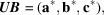which, when inverted, gives the vector components of the corresponding real-space cell. The elements of UB can be measured directly from the diffraction pattern in millimetres. Define axes x and y to be in the recording plane and z in the beam direction. A point in the diffraction pattern x, y, z is then related to the indices h, k, l by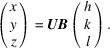Note that points with non-zero z are observed on the plane z = 0, see Fig. 5.4.1.1.Figure 5.4.1.1 | top | pdf |Diffraction geometry. Crystal at C with the direct transmitted beam, CRO, intersecting the reciprocal-lattice origin at R and the recording plane at normal incidence at O. The camera length L is CO and the reciprocal of the wavelength λ is CR.

The metric M of UB−1 is used to find the unit-cell edges and angles as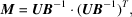where T means the transpose. Then,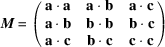gives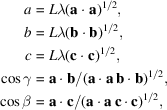and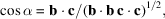where L is the effective distance between the diffracting crystal and the recording plane and λ is the wavelength. These quantities are defined in Fig. 5.4.1.1together with the nomenclature and geometrical relationships required in this article.

If necessary, the cell is reduced to the Bravais cell according to the procedures given in IT A (2005, Chapter 9.2), before calculating the metric.

In practice, there may be a difficulty in choosing a vector set that describes a primitive reciprocal cell. Although a record of any reasonably dense plane of reciprocal space immediately exposes two basis vectors of a cell, the third vector lies out of the plane of the diffraction pattern containing the first two vectors and may not be directly measurable. Hence, some care must be taken to ensure that the third vector chosen makes the cell primitive. This presents no difficulty in the non-zero-zone analysis given in Subsection 5.4.1.3but needs consideration when two patterns are used as described in Subsection 5.4.1.2.

Unit-cell parameters may be partly or fully determined depending on the extent of knowledge of the effective camera length L and wavelength λ, and upon the type and number of patterns. The different situations are distinguished in Table 5.4.1.1for photographic recording. Note that L is simply a magnification factor.

 Table 5.4.1.1| top | pdf | Unit-cell information available for photographic recording
Pattern typeConstants knownInformation available
(1) Zero zone None or λ or L d ratios and interplane angles
(2) Lλ or L and λ d values and interplane angles
(3) Multiple zone None or L As for (1)
(4) Lλ As for (2)
(5) λ Unit-cell axial ratios and angles
(6) L and λ Unit-cell axes and angles
(7) Two or more zero-zone patterns None or L As for (5)
(8) Lλ As for (6)

The accuracy of the non-zero-zone analysis described in Subsection 5.4.1.3will depend on the influence of spiral, radial, and elliptical distortions. The camera length and wavelength need to be known to better than 1%.

For measurements made using microscope deflector systems, a knowledge of L may not be required depending on the location of the deflectors in the microscope column. Instead, the calibration factor of the deflectors, in suitable units, will replace L.

#### 5.4.1.2. Zero-zone analysis

| top | pdf |

Two patterns are required that represent different sections through the reciprocal lattice. The angle of rotation p between these sections must be known, as well as the trace of the rotation axis in the plane of the pattern. Define the plane of the first pattern as the xy plane with the x axis, for convenience, coincident with the trace of the rotation axis. The coordinates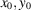of reflections in the first pattern are then measured. The coordinates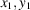of spots in the patterns rotated by p relative to the first pattern are then measured, care being taken to align the trace of the rotation axis with the x axis of the measuring equipment for each pattern. The coordinates of these reflections are then reduced to the coordinate system of the first pattern by the relations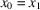,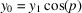,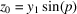. The coordinates of all reflections measured are placed in a table that is scanned to extract the three shortest non-coplanar vectors. If the patterns come from dense, neighbouring zones, it is likely that these vectors define a primitive cell.

#### 5.4.1.3. Non-zero-zone analysis

| top | pdf |

One pattern with well defined Laue zones taken at an arbitrary zone allows the recovery of a third vector not in the plane of the pattern. First find the components for the two shortest zero-zone vectors a* and b* (see Fig. 5.4.1.2). For the third vector, measure the x and y coordinates of a non-zero-zone vector. These are then transformed to components in the coordinate system defined by the two shortest vectors of the zero zone. Then the fractional part of each transformed component is taken. If the vector comes from a Laue zone of order n, these fractional parts must be divided by n. A transformation back to Cartesian coordinates gives the x and y components of the third vector c*, point O′ in Fig. 5.4.1.2. In practice, it is best to measure a set of vectors at roughly equal angles around the zone so that an average can be taken to improve accuracy.Figure 5.4.1.2 | top | pdf |A diffraction pattern with the crystal oriented at a zone axis. The lower diagram shows the zero-zone vectors a* and b*, and OO′, the projection on the xy plane of c*.

For certain space groups and special orientations, it is possible for half of the zero-zone reflections to be absent. If sufficient non-zero-zone vectors have been measured, two different vectors OO′ should be found. New axes (a* + b*)/2 and (a* − b*)/2 must then be chosen and the x and y coordinates of c* recalculated.

The z component of c* is obtained (Fig. 5.4.1.1) from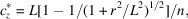where r is the radius of the Laue circle of order n.

We now have the orientation matrix UB as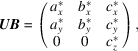and the measurement of the pattern is complete.

After transformation of the axes defined by UB to the Bravais axes, the inverse of the resulting UBBravais will index the pattern using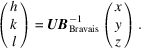### References

Edington, J. W. (1975). Electron diffraction in the electron microscope. Monographs in practical electron microscopy in materials science, No. 2. Eindhoven: N. V. Philips Gloeilampenfabrieken.
Gard, J. A. (1976). Electron microscopy in mineralogy, p. 52. Berlin: Springer.
Hirsch, P. B., Howie, A., Nicholson, R. B., Pashley, D. W. & Whelan, M. J. (1965). Electron microscopy of thin crystals. London: Butterworth.
International Tables for Crystallography (2005). Vol. A. Heidelberg: Springer.
LePage, Y. (1992). Ab initio primitive cell parameters from single convergent beam patterns: a converse route to the identification of microcrystals with electrons. Microsc. Res. Tech. 21, 158–165.
Zuo, J. M. (1993). New method of Bravais lattice determination. Ultramicroscopy, 52, 459–464.